# It works! Kids will master their math skills, having fun the whole time.

Our individualized learning program is designed based on developmental theory, evidenced-based early number interventions, and Maria Montessori’s early mathematics approach.## Here's how it works.

• When children begin playing Math Shelf, they take a placement test that assigns them to games and activities that match their knowledge and skill level.
• Teachers schedule students to play Math Shelf in class for 10 minutes, twice per week. Each student will receive sequenced and adaptive instruction.
• Math Shelf has over 1,500 games and activities to keep students engaged throughout the year.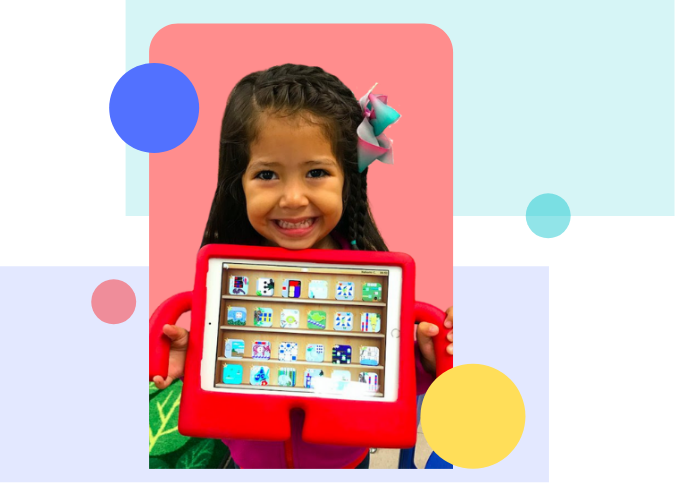## The Skills Math Shelf Teaches

Your Pre-K & Kindergarten students will learn a whole lot of math!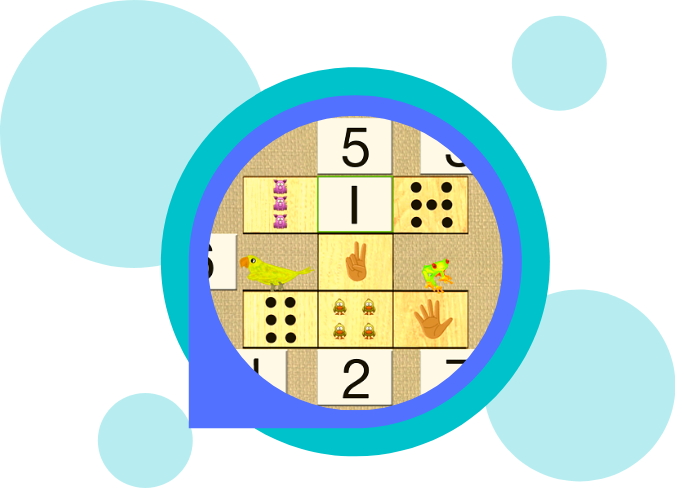### Pre-Math Skills

• Names and matches primary and secondary colors.
• Matches items by size, shape, and orientation
• Sorts objects into 2, 3, and 4 categories
• Orders up to 5 items from smallest to largest
• Counts with 1-to-1 correspondence
• Identifies similarities and differences
• Classifies objects

### Counting & Cardinality

• Counts to 100 by ones, fives, and tens
• Counts to answer "how many?" questions
• Understands that the last number name tells how many objects were counted
• Counts forward & backwards beginning from a given number to 100
• Writes numbers from 0 to 1,000
• Identifies whether the number of objects in one group is greater than, less than, or equal to the number of objects in another group
• Compares numbers between 1 and 100 when presented as written numerals
• Reads and writes numerals, and represents a number of objects with a written numeral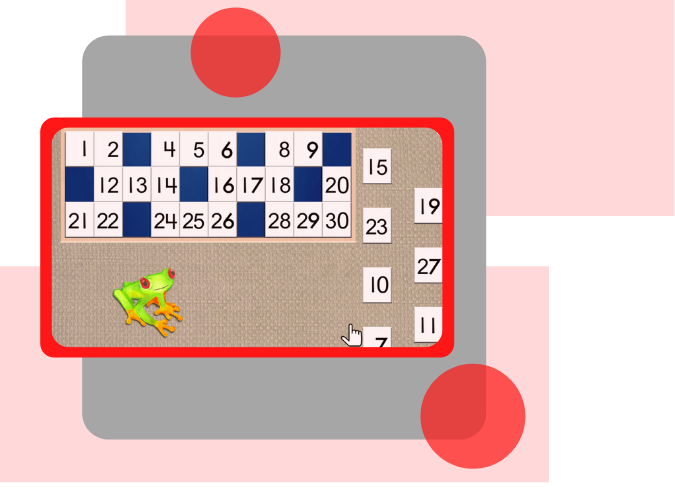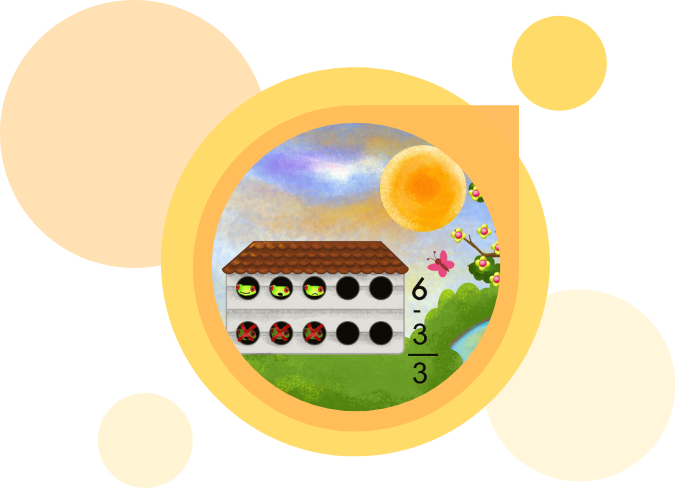### Operations & Algebraic Thinking

• Decompose numbers less than or equal to 10 into pairs in more than one way
• Represent addition and subtraction with objects, fingers, mental images, and drawings up to 10
• Fluently add and subtract within 10
• Solve addition and subtraction word problems within 20
• Add and subtract within 20 by using fluency facts for addition-subtraction within 10
• Understand the meaning of the equal sign, and determine if equations involving addition and subtraction are true or false
• Determine the unknown whole number in an addition or subtraction equation

### Numbers & Operations in Base Ten

• Compose and decompose numbers from 11 to 19 into tens and ones
• Understand place value of a three-digit number and can identify hundreds, tens and ones place
• Compare two, two-digit numbers using symbols >, =, <
• Given a two-digit number, mentally find 10 more or 10 less than the number
• Add within 100 using concrete models that represent place value
• Subtract multiples of 10 in the range 10-90 using concrete models, drawings, and place value strategies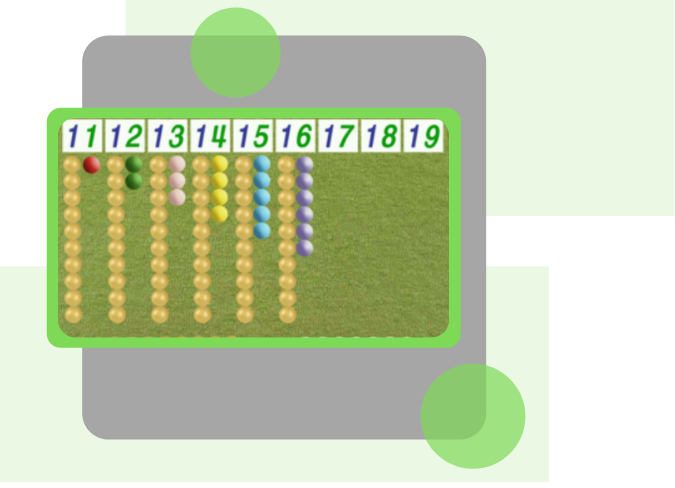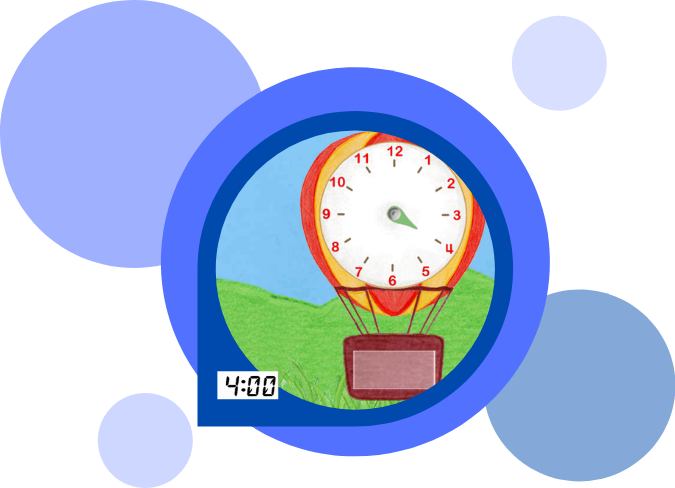### Measurement

• Weight. Measure and record the weight of various objects, and add smaller objects to calculate the weight of larger objects
• Temperature. Measure and record the temperature, and associate thermometer readings with seasons and weather
• Time. Tell and write time in hours and half-hours using analog and digital clocks
• Money. Solve problems involving dollar bills, quarters, dimes, nickels, and pennies, using \$ and ¢ symbols appropriately
• Length. Order objects by length; compare the lengths, and express the length of an object as a whole number of units
• Data. Organize, represent, and interpret data with up to three categories; ask and answer questions about how many more or less are in one category than in another

### Geometry

• Correctly name shapes regardless of their orientations or size
• Identify shapes as two-dimensional or three-dimensional
• Analyze and compare two- and three-dimensional shapes, in different sizes and orientations
• Identify and name shapes in one's environment
• Compose simple shapes to form larger shapes (2 triangles to form a rectangle, 3 triangles to form a trapezoid, 5 triangles to form a pentagon, etc.)
• Fractions. Partition circles and rectangles into two, three, four, five, and six equal shares. Describe shares using the words halves, thirds, fourths, etc.
• Count shape sides and angles and distinguish between different shape's defining attributes## 94% of students master the Common Core standards by playing Math Shelf just 20 minutes per week.

Math Shelf makes an impact.